Sample Data: Hamster Tumour

Locations of cell nuclei in hamster kidney annotated with type

Details

Locations of cell nuclei in hamster kidney in the observation region Rectangle[{0, 0}, {1, 1}]*250 microns, annotated with type.

Examples

Basic Examples (1)

 In:=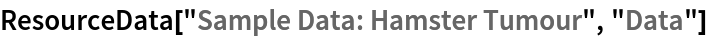Out=Summary of the spatial point data:

 In:=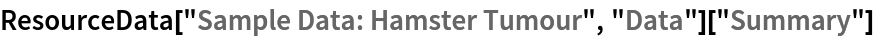Out=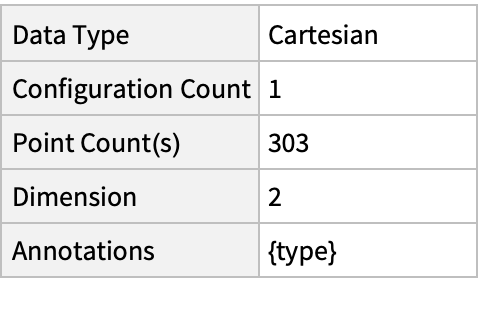Visualizations (2)

Plot the spatial point data:

 In:=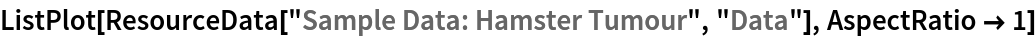Out=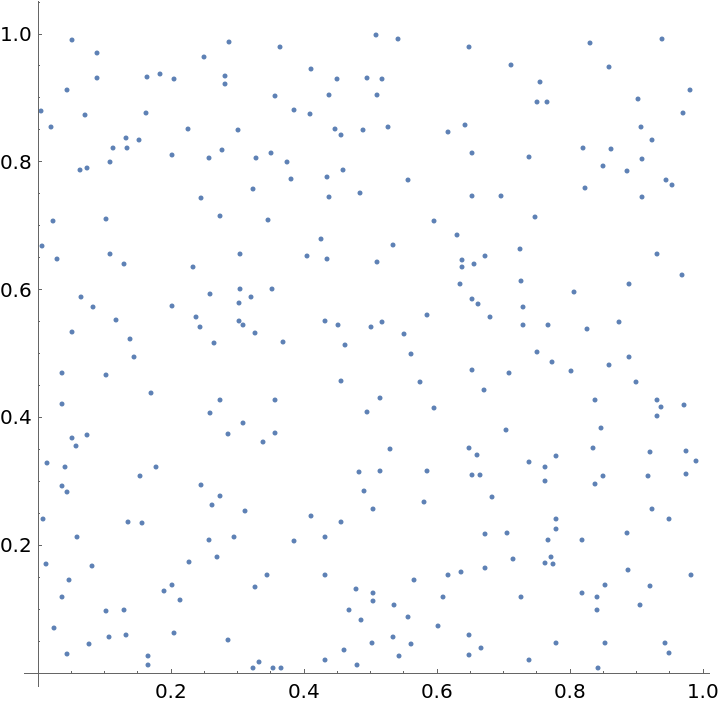Visualize points with the type annotations:

 In:=Out=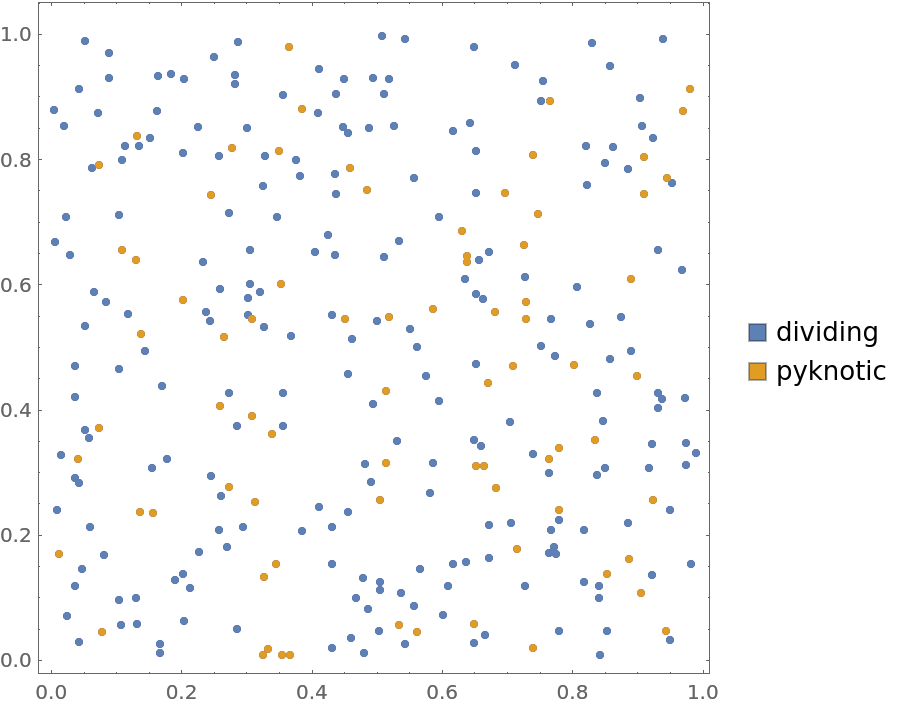Analysis (5)

Compute probability of finding a point within given radius of an existing point - NearestNeighborG is the CDF of the nearest neighbor distribution:

 In:=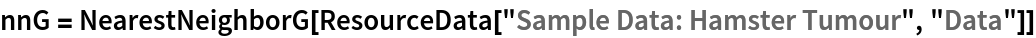Out=In:=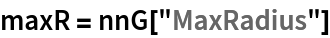Out=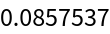In:=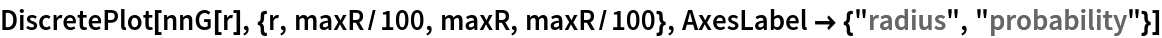Out=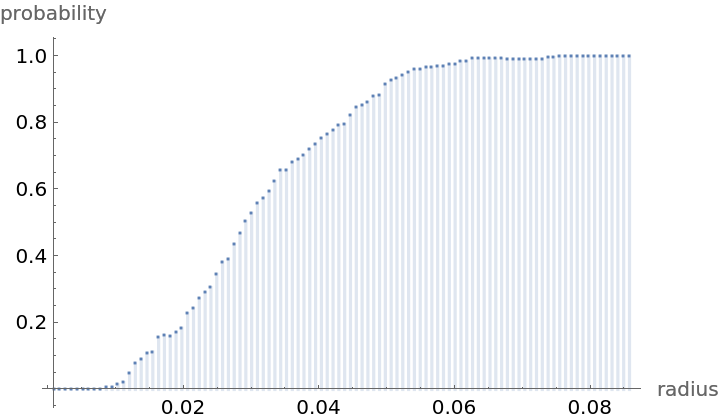Mean distance between a typical point and its nearest neighbor (for positive support distribution can be approximated via a Riemann sum of 1-CDF):

 In:=In:=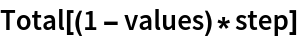Out=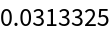Conform to scale:

 In:=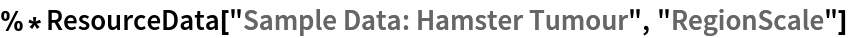Out=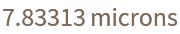Test for complete spacial randomness:

 In:=Out=Fit a Poisson point process to data:

 In:=Out=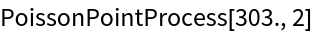Gosia Konwerska, "Sample Data: Hamster Tumour" from the Wolfram Data Repository (2021)